Get instant live expert help with Excel or Google Sheets“My Excelchat expert helped me in less than 20 minutes, saving me what would have been 5 hours of work!”

#### Post your problem and you’ll get Expert help in seconds.

Your message must be at least 40 characters
Our professional Expert are available now. Your privacy is guaranteed.

# How to Remove a Trailing Slash from a URL Link in Excel

We can remove a trailing slash from a URL LINK by using a combination of LEFT, RIGHT, and LEN functions. This will delete the slash from the URL. We will walk through the steps below to understand how this works.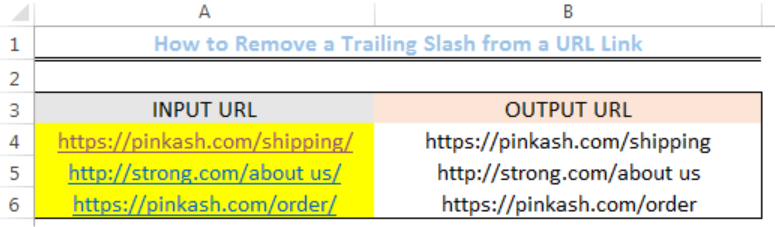Figure 1: Results of removed slash from URL using the LEFT, RIGHT, and LEN functions

## Formula

`=LEFT(A4,LEN(A4)-(RIGHT(A4)="/"))`

## Setting up the Data

• We will set up our data by inputting 3 URLs in Cell A4 to Cell A6
• We will name Cell B3 as OUTPUT URL and Cell A3 as INPUT URL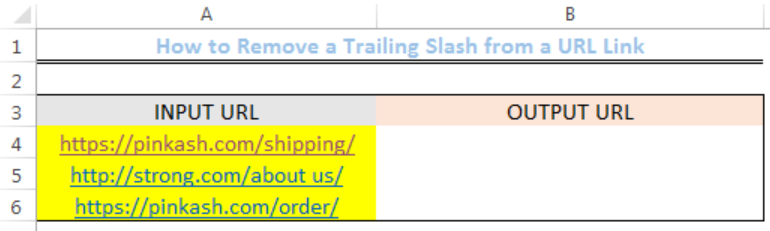Figure 2: How to Remove a Trailing Slash from a URL Link

## Removing a Trailing Slash from a URL Link

• We will click on Cell B4 and then, formulas on the menu bar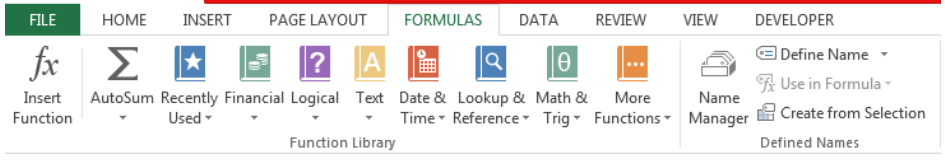Figure 3: How to Remove a Trailing Slash from a URL Link

• We will click on Text, scroll down the list and click on LEFT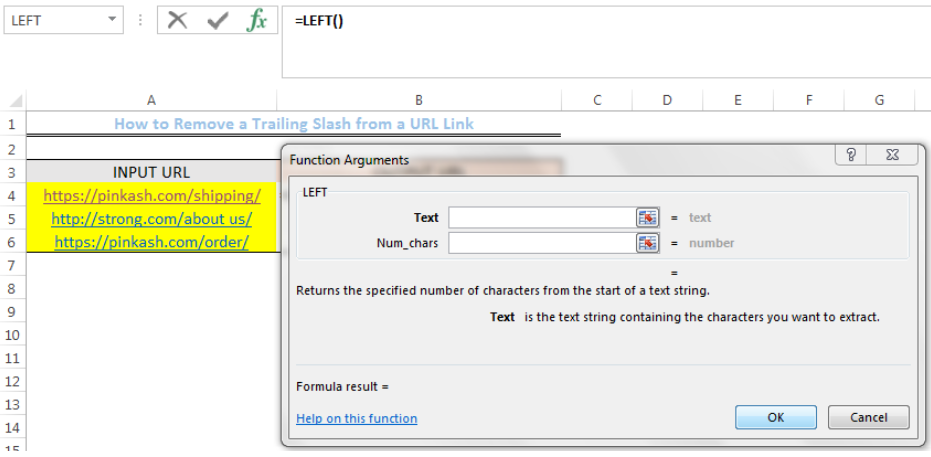Figure 4: How to Remove a Trailing Slash from a URL Link

• We will see the dialog box as shown in figure 4
• We will input the formula below into the text field

`(A4,LEN(A4)-(RIGHT(A4)="/"))`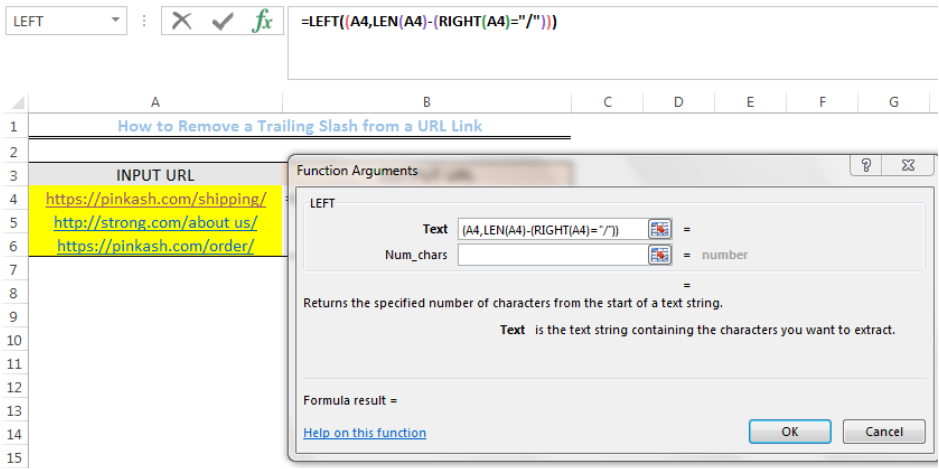Figure 5: How to Remove a Trailing Slash from a URL Link

• We will click OK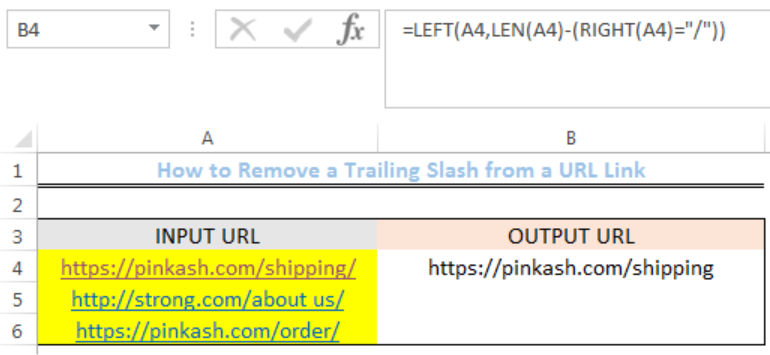Figure 6: How to Remove a Trailing Slash from a URL Link

• We will use the drop-down feature to get the OUTPUT URL of Cell A5 and Cell A6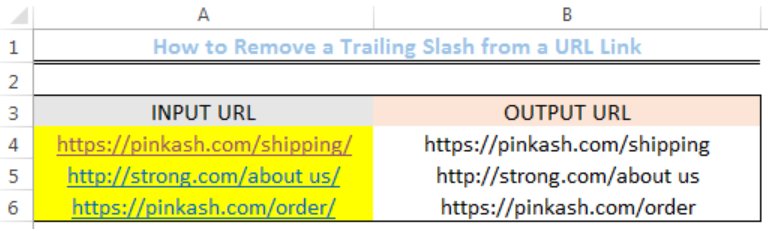Figure 7: Results of removed slash from URL using the LEFT, RIGHT, and LEN functions

## Note

We can also remove the trailing slash by typing the formula below into Cell B4 and then hit the enter button.

`=LEFT(A4,LEN(A4)-(RIGHT(A4)="/"))`

## Explanation

• Trailing slash (“/”)

This is the last character that appears in each of the INPUT URL

• The formula `=LEFT(A4,LEN(A4)-(RIGHT(A4)="/"))`

This formula is used to extract all values to the left in the specified cell. It, however, removes the last character from the URL PROVIDED IT IS A SLASH. Hence, if a URL doesn’t contain a slash at the end, the output will be the same as the input.

## Instant Connection to an Expert through our Excelchat Service

Most of the time, the problem you will need to solve will be more complex than a simple application of a formula or function. If you want to save hours of research and frustration, try our live Excelchat service! Our Excel Experts are available 24/7 to answer any Excel question you may have. We guarantee a connection within 30 seconds and a customized solution within 20 minutes.

### Did this post not answer your question? Get a solution from connecting with the expert.Another blog reader asked this question today on Excelchat:
Solution examplesWhat is the formula for splitting up one column text into 2 columns?
Solved by B. H. in 20 minsI have text and number in multiple cells (Ex: Cell 1: 4S, Cell 2: 3.5A, Cell 3: 8O) and want to sum the number values only (Ex: 4 + 3.5 + 8). How can I do this?
Solved by O. A. in 60 minsI am having issues creating the one way data table to show the means and standard deviations when you change the proportion of portfolio 1. I have the mean formula and the standard deviation formula at the top and -1 through 1 down the left side. I highlight all of it and create a data table. I reference the "proportion of portfolio 1" cell in the column input box but when the data table comes out it has all the same numbers all they way down the table.
Solved by G. Q. in 49 minsI need to create a formula that extracts the prefix from column A
Solved by I. Q. in 42 minsI have 2 columns one of them with a dropdown list of 20 and the other one with 2 options only. I want that when Comment A and B are chosen in the first column then the secon column populate "X" STRAIGHT AWAY. HOW DO i DO IT?
Solved by I. D. in 10 mins## Subscribe to Excelchat.coAnother blog reader asked this question today on Excelchat: